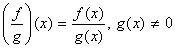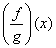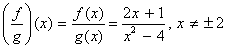# Difference between f(x) and g(x)

Functions with overlapping domains can be added, subtracted, multiplied, and divided. If   (   ) and   (   ) are two functions, then for all   in the domain of both functions the sum, difference, product and quotient are defined as follows.

(  f + g  )(   ) =   (   ) +   (   )

(  f – g  )(   ) =   (   ) –   (   )

(  fg  )(   ) =   (   ) ×   (   )Let’s go with an example:

Let’s say   (   ) = 2   + 1 and   (   ) =  x   – 4.

Find (  f + g  )(   ), (  f – g  )(   ), (  fg  )(   ) and.

(  f + g  )(   ) =   (   ) +   (   )

= (  2x  + 1) + (  x2  –  )

=  x2  +  2x  –

(  f – g  )(   ) =   (   ) –   (   )

= (  2x  + 1) – (  x2  –  )

= –  x2  +  2x   5

(  fg  )(   ) =   (   ) ×   (   )

= (  2x  + 1)(  x2  –  )

=  2×3  +  x2  –  8x  – 4There is another way to combine two functions to create a new function is called function composition. In function composition we substitute an entire function into another function.

The notation of the function f with g is and is read f of g of x . It means that wherever there is an x ​​in the function f , it is replaced with the function g ( x ). The domain of is the set of all x’s in the domain of g such that g(x) is in the domain of f.

Example 1:

Say f ( x ) = x 2 and g ( x ) = x – 3. Find f ( g ( x )).

Example 2:

Say f ( x ) = 2 x – 1 and g ( x ) = x + 2. Find f ( g ( x )).

Note : The order IF matters when you find the composition of functions.